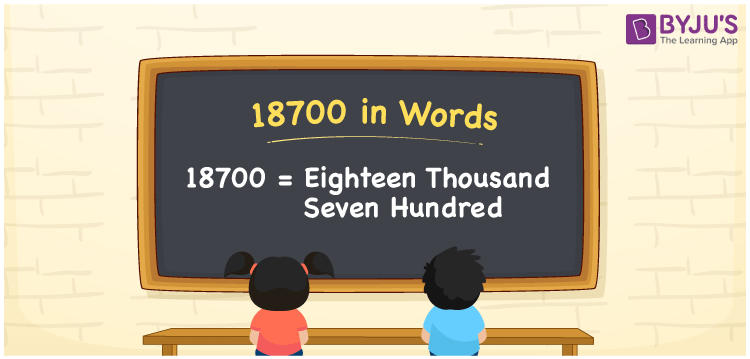# 18700 in Words

18700 in words is written as Eighteen thousand seven hundred. In both the International System of Numerals and the Indian System of Numerals, 18700 is written as Eighteen thousand seven hundred. The number 18700 is a Cardinal Number as it represents some quantity. For example, “that smartphone costs 18700 rupees”.

 18700 in Words Eighteen thousand seven hundred Eighteen thousand seven hundred in Number 18700

## 18700 in English Words

18700 in English words is read as “Eighteen thousand seven hundred.”## How to Write 18700 in Words?

To write 18700 in words, we shall use the place value chart. In the place value chart, put 1 in the ten thousands, 8 in the thousands, 7 in the hundreds, and 0 in the tens and the ones, respectively. Let us make a place value chart to write the number 18700 in words.

 Ten Thousands Thousands Hundreds Tens Ones 1 8 7 0 0

Thus, we can write the expanded form as

1 × Ten Thousand + 8 × Thousand + 7 × Hundred + 0 × Ten + 0 × One

= 1 × 10000 + 8 × 1000 + 7 × 100 + 0 × 10 + 0 × 1

= 10000 + 8000 + 700 + 0 + 0

= 18700

= Eighteen thousand seven hundred.

18700 is the natural number which is the successor of 18699 and predecessor of 18701.

18700 in words – Eighteen thousand seven hundred

• Is 18700 an odd number? – No
• Is 18700 an even number? – Yes
• Is 18700 a perfect square number? – No
• Is 18700 a perfect cube number? – No
• Is 18700 a prime number? – No
• Is 18700 a composite number? – Yes

## Frequently Asked Questions on 18700 in Words

Q1

### How to write 18700 in words?

18700 in words is written as Eighteen thousand seven hundred.
Q2

### How to write 18700 in words in the International and Indian System of Numerals?

In both, the system of numerals, 18700 in words, is written as Eighteen thousand seven hundred.
Q3

### How to write 18700 in a place value chart?

In the place value chart, write 1 in the ten thousands, 8 in the thousands, 7 in the hundreds and 0 in the tens and ones, respectively.